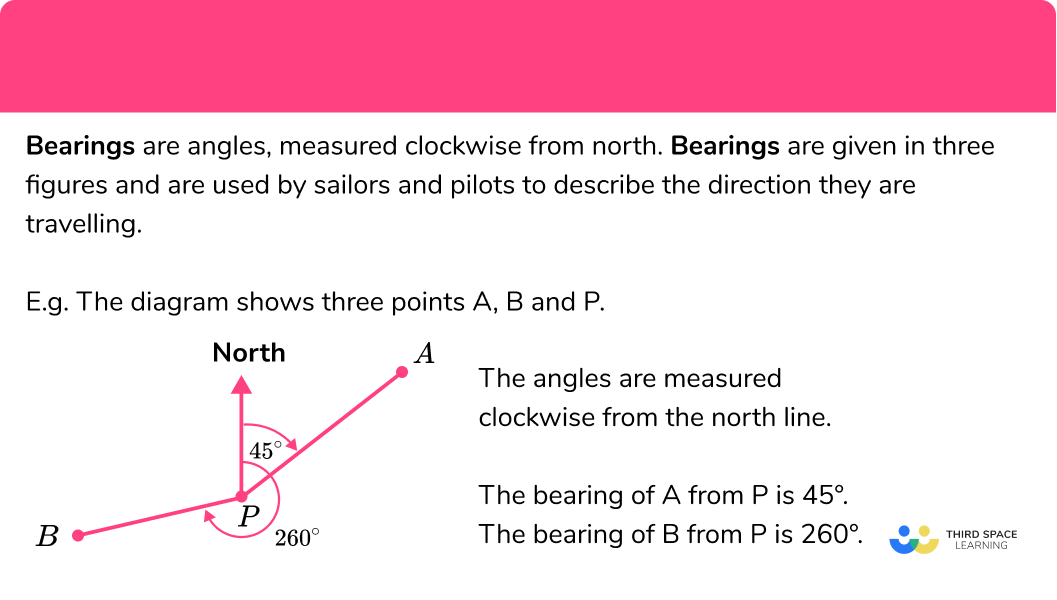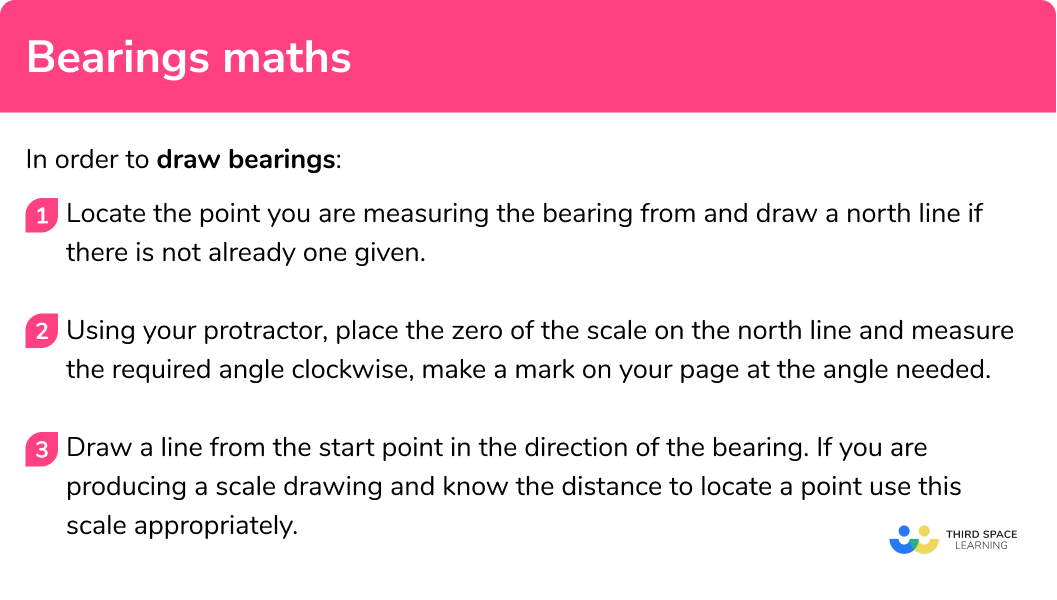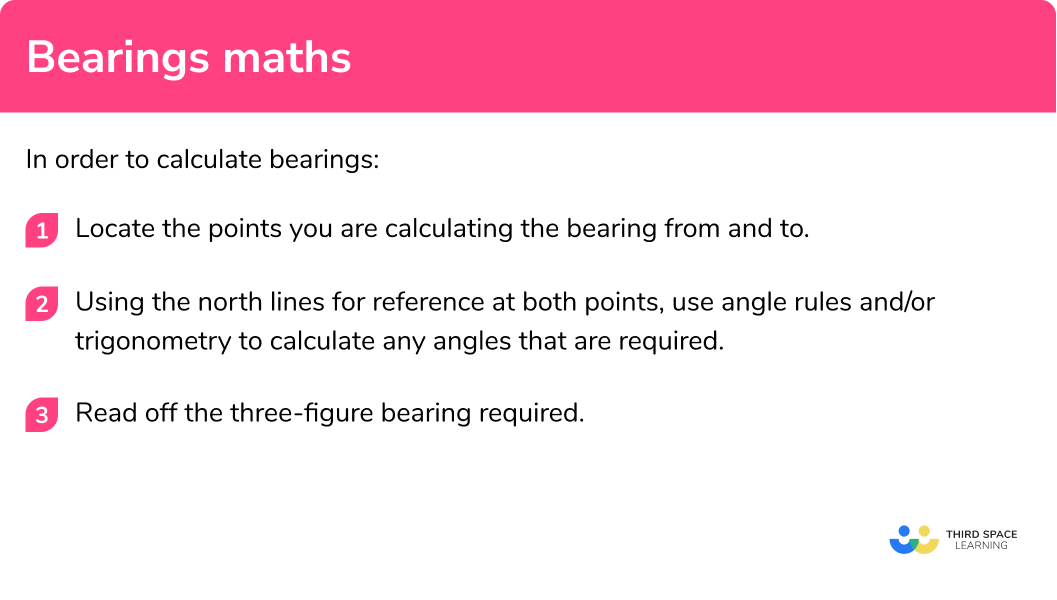# Bearings

Here we will learn about bearings, including measuring bearings, drawing bearings and calculating bearings.

There are also bearings maths worksheets based on Edexcel, AQA and OCR exam questions, along with further guidance on where to go next if you’re still stuck.

## What are bearings?

Bearings are angles, measured clockwise from north.

To measure a bearing, we must first know which direction is north.

This north direction is usually provided in the maths exam question. We then measure the required angle in a clockwise direction. All bearings need to be given in three figures, so if the angle measured is less than 100 degrees, we must start the three-figure bearing with a zero.

Example:

The diagram shows three points A, B and P.

The angles are measured clockwise from the north line.

The bearing of A from P is 045^{\circ}

The bearing of B from P is 260^{\circ} .

Bearings are used by sailors and pilots to describe the direction they are travelling. They are also used on land by hikers and the military.

### What are bearings?## How to draw bearings

In order to draw bearings:

1. Locate the point you are measuring the bearing from and draw a north line if there is not already one given.
2. Using your protractor, place the zero of the scale on the north line and measure the required angle clockwise, make a mark on your page at the angle needed.
3. Draw a line from the start point in the direction of the bearing. If you are producing a scale drawing and know the distance to locate a point use this scale appropriately.

### Explain how to draw bearings### Related lessons on loci and constructions

Bearings is part of our series of lessons to support revision on loci and construction. You may find it helpful to start with the main loci and construction lesson for a summary of what to expect, or use the step by step guides below for further detail on individual topics. Other lessons in this series include:

## Bearings maths examples (drawing)

### Example 1: drawing a bearing less than 180°

Draw a bearing of 050^{\circ}

1. Locate the point you are measuring the bearing from and draw a north line if there is not already one given.

2Using your protractor, place the zero of the scale on the north line and measure the required angle clockwise, make a mark on your page at the angle needed.

3Draw a line from the start point in the direction of the bearing. If you are producing a scale drawing and know the distance to locate a point use this scale appropriately.

### Example 2: drawing a bearing more than 180°

Draw a bearing of 300^{\circ}

If you have a 180^{\circ} protractor, we need to subtract the bearing required from 360^{\circ}

360^{\circ} - 300^{\circ} = 60^{\circ} , so measure 60^{\circ} anticlockwise

### Example 3: drawing a scale drawing with a bearing

Make a scale drawing of a point Q 8 km away from a point P on a bearing of 110^{\circ} from P, using a scale of 1 cm : 2 km.

If the angle is more than 180^{\circ} and you do not have a full circle protractor then you should subtract the required angle from 360^{\circ} , and then measure this angle anticlockwise from the north line.

If the scale is 1cm : 2km , we will need to measure 4 cm to locate Q.

## How to calculate bearings

In order to calculate bearings:

1. Locate the points you are calculating the bearing from and to.
2. Using the north lines for reference at both points, use angle rules and/or trigonometry to calculate any angles that are required.
3. Read off the three-figure bearing required.

### How to calculate bearings## Bearings maths examples (calculating)

### Example 1: calculating a bearing around a point

Calculate the bearing of A from P.

We need the angle around the point P clockwise from the north line. We know that angles on a straight line add to 180^{\circ}. 180^{\circ} + 53^{\circ} = 233^{\circ}

The bearing of A from P is 233^{\circ} .

### Example 2: calculating a back bearing

The bearing of B from A is 070^{\circ} . Calculate the bearing of A from B.

Drawing a north line at B and extending the line from A to B shows us a corresponding angle. If we travel from A to B, we need to turn another 180^{\circ} to return back to A. 180^{\circ} + 70^{\circ} = 250^{\circ}

The bearing of A from B is 250^{\circ} .

### Example 3: calculate a bearing using SOHCAHTOA

A ship sails 7 km due east from a point P to point A. It then sails 3 km due south from A to point B. Calculate the bearing of B from P.

Sketch the diagram to help visualise the problem.

Drawing a line from P to B forms a right angled triangle. Use trigonometry to find the angle APB. Then add this to 90^{\circ} .

{{\tan }^{-1}}\left( \frac{3}{7} \right)=23^{\circ} to the nearest degree (bearings are normally given to the nearest degree)

90^{\circ} +23{}^\circ =113^{\circ}

The bearing of B from P is 113^{\circ} .

### Common misconceptions

• Not giving bearings less than 100^{\circ} in three figures

E.g.

A bearing with an angle of 45^{\circ} must be given as 045^{\circ} .

• Using the anticlockwise angle as the bearing

A common error is to use the anticlockwise angle as the bearing.

E.g.

The bearing of B from A is 080^{\circ} , a common mistake would be to use the co-interior angle as the back bearing of A from B as 100^{\circ} , calculating clockwise, the bearing of A from B should be 260^{\circ} .

### Practice bearings maths questions

1. Which of the diagrams shows a bearing of 040^{\circ} ?Angle should be measured clockwise from north.

2. Which of the diagrams shows a bearing of 290^{\circ} ?Angle should be measured clockwise from north.

3. What bearing describes due east?

090^{\circ}90^{\circ}270^{\circ}180^{\circ}north is 000^{\circ} , east is 090^{\circ} , west is 270^{\circ} and south is 180^{\circ} .

4. Calculate the bearing of A from P.125^{\circ}055^{\circ}305^{\circ}235^{\circ}Angle should be measured clockwise from north, so subtract 125^{\circ} from 360^{\circ} .

5. The bearing of C from B is 130^{\circ} . Calculate the bearing of B from C.

050^{\circ}310^{\circ}130^{\circ}230^{\circ}130^{\circ} + 180^{\circ} = 310^{\circ}

6. A boat sails 8 km north from P to Q and then sails 6 km west from Q to R. Calculate the bearing of R from P. Give your answer to the nearest degree.

037^{\circ}053^{\circ}217^{\circ}323^{\circ}Sketch a diagram. Angle should be measured clockwise from north, so find the angle QPR using trigonometry and subtract from 360^{\circ} .### Bearings maths GCSE questions

1. The bearing of A from B is 215^{\circ} . Find the bearing of B from A.

(2 marks)

Either 215 – 180 or 360 – 215 = 145 seen

(1)

035^{\circ}

(1)

2. The point C is on a bearing of 065^{\circ} from point A and on a bearing of 310^{\circ} from point B.

On the diagram, mark with a (x) the position of point C.(2 marks)

Correct line from A or correct line from B

(1)

Both lines correct and (x) shown

(1)3. The diagram shows the positions of three twins labeled P, Q and R.

Q is on a bearing of 080^{\circ} from P.

R is on a bearing of 132^{\circ} from P.

The distance PQ is 15 km and the distance PR is 14 km.a) Find the distance QR

b) Find the bearing of R from Q

(8 marks)

a)

Angle QPR = 132^{\circ}- 80^{\circ}= 52^{\circ}

(1)

Values substituted into cosine rule QR ^2=15^2+14^2-2 \times 15 \times 14 \times \cos 52

(1)

QR = 12.74 km

(1)

b)

Use of sine rule \frac{\sin{52}}{12.74}=\frac{\sin{PQR}}{14}

(1)

Angle PQR = 59.96^{\circ}

(1)

Angle of 100^{\circ} anticlockwise from north line at Q

(1)

360-100-59.96

(1)

(1)

## Learning checklist

You have now learned how to:

• Measure line segments and angles in geometric figures, including interpreting maps and scale drawings and use of bearings

## Still stuck?

Prepare your KS4 students for maths GCSEs success with Third Space Learning. Weekly online one to one GCSE maths revision lessons delivered by expert maths tutors.

Find out more about our GCSE maths tuition programme.